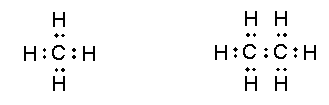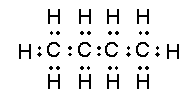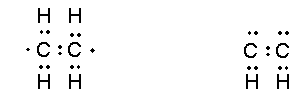Elements and Compounds

# Hydrogen to Carbon

Hydrogen to Carbon

Many of the most important chemical fuels are compounds composed entirely of carbon andhydrogen, i.e. hydrocarbons. The smallest of these is methane CH4, a primary component of household natural gas. Other simple common fuels include ethane C2H6, propane C3H8butaneC4H10, pentane C5H12, hexane C6H14, heptane C7H16, and octane C8H18. It is interesting to note that there is a consistency in these molecular formulae: in each case, the number of hydrogen atoms is two more than twice the number of carbon atoms, so that each compound has a molecular formula like CnH2n+2. This suggests that there are strong similarities in the valences of the atoms involved which should be understandable in terms of our valence shell electron pair sharing model. In each molecule, the carbon atoms must be directly bonded together, since they cannot be joined together with a hydrogen atom. In the easiest example of ethane, the two carbon atoms are bonded together, and each carbon atom is in turn bonded to three hydrogen atoms. Thus, in this case, it is relatively apparent that the valence of each carbon atom is 4, just as in methane, since each is bonded to four other atoms. Therefore, by sharing an electron pair with each of the four atoms to which it is bonded, each carbon atom has a valence shell of eight electrons.

In most other cases, it is not so trivial to determine which atoms are bonded to which, as there may be multiple possibilities which satisfy all atomic valences. Nor is it trivial, as the number of atoms and electrons increases, to determine whether each atom has an octet of electrons in its valence shell. We need a system of electron accounting which permits us to see these features more clearly. To this end, we adopt a standard notation for each atom which displays the number of valence electrons in the unbonded atom explicitly. In this notation, carbon and hydrogen look like figure 1, representing the single valence electron in hydrogen and the four valence electrons in carbon.Figure 1.

Using this notation, it is now relatively easy to represent the shared electron pairs and the carbon atom valence shell octets in methane and ethane. Linking bonded atoms together and pairing the valence shell electrons from each gives figure 2.Figure 2.

Recall that each shared pair of electrons represents a chemical bond. These are examples of what are called Lewis structures, after G.N. Lewis who first invented this notation. These structures reveal, at a glance, which atoms are bonded to which, i.e. the structural formula of the molecule. We can also easily count the number of valence shell electrons around each atom in the bonded molecule. Consistent with our model of the octet rule, each carbon atom has eight valence electrons and each hydrogen has two in the molecule.

In a larger hydrocarbon, the structural formula of the molecule is generally not predictable from the number of carbon atoms and the number of hydrogen atoms, so the molecular structure must be given to deduce the Lewis structure and thus the arrangement of the electrons in the molecule. However, once given this information, it is straightforward to create a Lewis structure for molecules with the general molecular formula CnH2n+2 such as propane, butane, etc. For example, the Lewis structure for “normal” butane (with all carbons linked one after another) is found here.Figure 3.

It is important to note that there exist no hydrocarbons where the number of hydrogens exceeds two more than twice the number of carbons. For example,

CH5 does not exist, nor does C2H8. We correspondingly find that all attempts to draw Lewis structures which are consistent with the octet rule will fail for these molecules. Similarly, CH3and C2H5 are observed to be so extremely reactive that it is impossible to prepare stable quantities of either compound. Again we find that it is not possible to draw Lewis structures for these molecules which obey the octet rule.

We conclude from these examples that, when it is possible to draw a Lewis structure in which each carbon has a complete octet of electrons in its valence shell, the corresponding molecule will be stable and the hydrocarbon compound will exist under ordinary conditions. After working a few examples, it is apparent that this always holds for compounds with molecular formula CnH2n+2.

On the other hand, there are many stable hydrocarbon compounds with molecular formulae which do not fit the form CnH2n+2, particularly where the number of hydrogens is less than 2n+2. In these compounds, the valences of the carbon atoms are not quite so obviously satisfied by electron pair sharing. For example, in ethene C2H4 and acetylene C2H2 there are not enough hydrogen atoms to permit each carbon atom to be bonded to four atoms each. In each molecule, the two carbon atoms must be bonded to one another. By simply arranging the electrons so that the carbon atoms share a single pair of electrons, we wind up with rather unsatisfying Lewis structures for ethene and acetylene, shown here.Figure 4.

Note that, in these structures, neither carbon atom has a complete octet of valence shell electrons. Moreover, these structures indicate that the carbon-carbon bonds in ethane, ethene, and acetylene should be very similar, since in each case a single pair of electrons is shared by the two carbons. However, these bonds are observed to be chemically and physically very different. First, we can compare the energy required to break each bond (the bond energy or bond strength). We find that the carbon-carbon bond energy is 347 kJ in C2H6, 589 kJ in C2H4, and 962 kJ in C2H2. Second, it is possible to observe the distance between the two carbon atoms, which is referred to as the bond length. It is found that carbon-carbon bond length is 154 pm in C2H6, 134 pm in C2H4, and 120 pm in C2H2. (1 picometer = 1 pm = 10-12m). These observations reveal clearly that the bonding between the carbon atoms in these three molecules must be very different.

Note that the bond in ethene is about one and a half times as strong as the bond in ethane; this suggests that the two unpaired and unshared electrons in the ethene structure above are also paired and shared as a second bond between the two carbon atoms. Similarly, since the bond in acetylene is about two and a half times stronger than the bond in ethane, we can imagine that this results from the sharing of three pairs of electrons between the two carbon atoms. These assumptions produce the Lewis structures here.Figure 5.

These structures appear sensible from two regards. First, the trend in carbon-carbon bond strengths can be understood as arising from the increasing number of shared pairs of electrons. Second, each carbon atom has a complete octet of electrons. We refer to the two pairs of shared electrons in ethene as a double bond and the three shared pairs in acetylene as a triple bond.

We thus extend our model of valence shell electron pair sharing to conclude that carbon atoms can bond by sharing one, two, or three pairs of electrons as needed to complete an octet of electrons, and that the strength of the bond is greater when more pairs of electrons are shared. Moreover, the data above tell us that the carbon-carbon bond in acetylene is shorter than that in ethene, which is shorter than that in ethane. We conclude that triple bonds are shorter than double bonds which are shorter than single bonds.